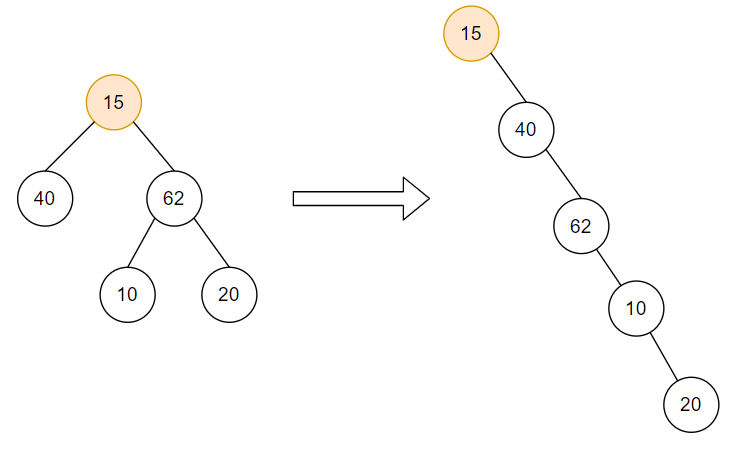# Flatten Binary Tree to Linked List

Posted: 28 Jan, 2021
Difficulty: Moderate

## PROBLEM STATEMENT

#### You are given a binary tree consisting of integer values. Your task is to convert the given binary tree into a linked list where the nodes of the linked list follow the same order as the pre-order traversal of the given binary tree.

##### Note:
``````Use the right pointer of the binary tree as the “next” pointer for the linked list and set the left pointer to NULL.
``````
``````Can you solve it using constant extra space?
``````
##### Example:``````Consider the binary tree rooted at 15, as shown above (left). On flattening the tree into a linked list we get the resulting tree, as shown above (right). Note that the linked list follows the same order as the pre-order traversal of the given binary tree.
``````
##### Input format:
``````The first line of input contains an integer 'T' denoting the number of queries or test cases.

The first and the only line of every test case contains elements of the binary tree in the level order form. The line consists of values of nodes separated by a single space. In case a node is null, we take -1 on its place.
``````
##### For example:
``````The input for the tree depicted in the below image would be:
````````````1
2 3
4 -1 5 6
-1 7 -1 -1 -1 -1
-1 -1
``````

#### Explanation :

``````Level 1 :
The root node of the tree is 1

Level 2 :
Left child of 1 = 2
Right child of 1 = 3

Level 3 :
Left child of 2 = 4
Right child of 2 = null (-1)
Left child of 3 = 5
Right child of 3 = 6

Level 4 :
Left child of 4 = null (-1)
Right child of 4 = 7
Left child of 5 = null (-1)
Right child of 5 = null (-1)
Left child of 6 = null (-1)
Right child of 6 = null (-1)

Level 5 :
Left child of 7 = null (-1)
Right child of 7 = null (-1)

The first not-null node(of the previous level) is treated as the parent of the first two nodes of the current level. The second not-null node (of the previous level) is treated as the parent node for the next two nodes of the current level and so on.
The input ends when all nodes at the last level are null(-1).
``````
##### Note :
``````The above format was just to provide clarity on how the input is formed for a given tree.
The sequence will be put together in a single line separated by a single space. Hence, for the above-depicted tree, the input will be given as:

1 2 3 4 -1 5 6 -1 7 -1 -1 -1 -1 -1 -1
``````
##### Output format:
``````For each test case, the nodes of the linked list, each separated by a single space, are printed.

Output for each test case is printed on a separate line.
``````
##### Note:
``````You do not need to print anything, it has already been taken care of. Just return the head of the linked list.
``````
##### Constraints:
``````1 <= T <= 100
1 <= No. of Nodes <= 5 * 10^4
1 <= data <= 10^5, data!=-1

Where 'data' is the integer data stored in a 'TreeNode' pointer.

Time limit: 1 sec
``````Approach 1
• A brute approach to solve this problem is by iterating over the tree in the pre-order fashion.
• While traversing, store the values of the nodes in a list.
• Create the required linked list using the stored values.